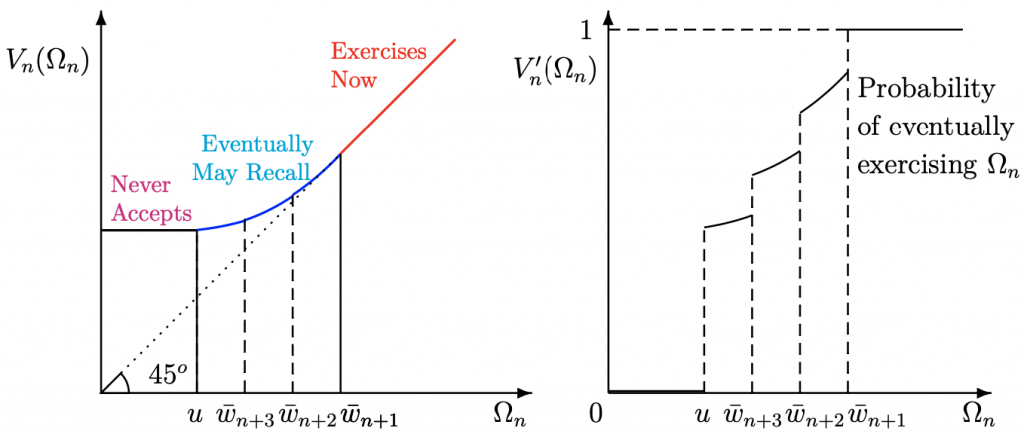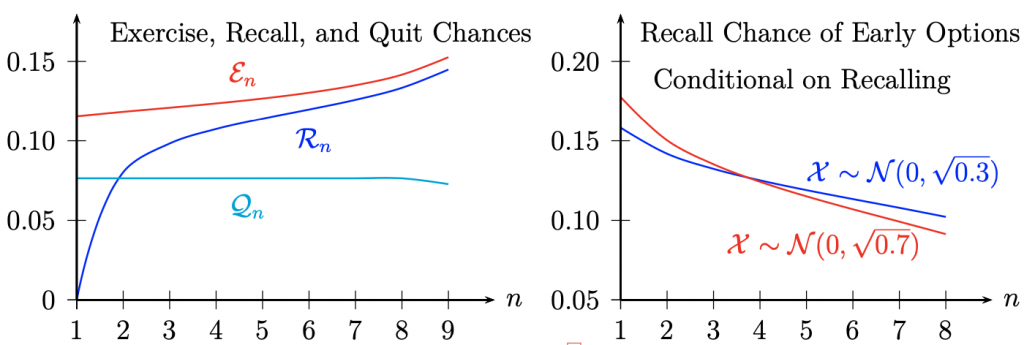# Optimal Sequential Search

We introduce a simple new model of sequential search among finitely many options that fits many economic applications. Each payoff is the sum of a random “known factor” and a “hidden factor”, learned at cost. Weitzman (1979) solved the ex post Pandora’s box problem, given known factors. Ours is the ex ante model for estimation, unconditional on known factors, and so resolves major selection effects.1. Search intensifies over time, as one increasingly exercises the current option, recalls a prior one, or quits. If one recalls, earlier options are recalled more often.2. We solve a long open question in all search models: which stochastic changes lead one to search longer? Answer: more dispersed payoffs.

3. The stationary search model poorly approximates search with many options: If the known factor density lacks a thin tail (eg. exponential), the recall chance is boundedly positive with vastly many options.

4. Search lasts longer with more options. Hence, if low search frictions increase worker applicant pools of firms, vacancy duration increases.